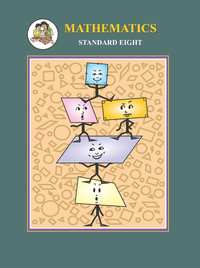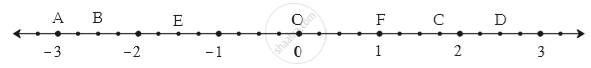SSC (English Medium) Class 8Maharashtra State Board
Share

# Balbharati solutions for Class 8 Mathematics chapter 1 - Rational and Irrational numbers

#### Balbharati Balbharati Class 8 Mathematics## Chapter 1: Rational and Irrational numbers

Practice Set 1.1Practice Set 1.2Practice Set 1.3Practice Set 1.4

#### Chapter 1: Rational and Irrational numbers Exercise Practice Set 1.1 solutions [Page 2]

Practice Set 1.1 | Q 1.1 | Page 2

Show the following numbers on a number line. Draw a separate number line for each example.

3/2 , 5/2 , -3/2

Practice Set 1.1 | Q 1.2 | Page 2

Show the following numbers on a number line. Draw a separate number line for each example.

7/5 , (-2)/5 , (-4)/5

Practice Set 1.1 | Q 1.3 | Page 2

Show the following numbers on a number line. Draw a separate number line for each example.

(-5)/8 , 11/8

Practice Set 1.1 | Q 1.4 | Page 2

Show the following numbers on a number line. Draw a separate number line for each example.

13/10 , (-17)/10

Practice Set 1.1 | Q 2 | Page 2

Observe the number line and answer the questions.(1) Which number is indicated by point B?

(2) Which point indicates the number 1 3/4 ?

(3) State whether the statement, ‘the point D denotes the number 5/2’ is true or false.

#### Chapter 1: Rational and Irrational numbers Exercise Practice Set 1.2 solutions [Page 3]

Practice Set 1.2 | Q 1 | Page 3

Compare the following number.

-7,-2

Practice Set 1.2 | Q 2 | Page 3

Compare the following number.

0,(-9)/5

Practice Set 1.2 | Q 3 | Page 3

Compare the following number.

8/7,0

Practice Set 1.2 | Q 4 | Page 3

Compare the following number.

(-5)/4,1/4

Practice Set 1.2 | Q 5 | Page 3

Compare the following number.

40/29,141/29

Practice Set 1.2 | Q 6 | Page 3

Compare the following number.

-17/20,(-13)/20

Practice Set 1.2 | Q 7 | Page 3

Compare the following number.

15/12,7/16

Practice Set 1.2 | Q 8 | Page 3

Compare the following number.

(-25)/8,(-9)/4

Practice Set 1.2 | Q 9 | Page 3

Compare the following number.

12/15,3/5

Practice Set 1.2 | Q 10 | Page 3

Compare the following number.

(-7)/11,(-3)/4

#### Chapter 1: Rational and Irrational numbers Exercise Practice Set 1.3 solutions [Page 4]

Practice Set 1.3 | Q 1 | Page 4

Write the following rational number in decimal form.

9/37

Practice Set 1.3 | Q 2 | Page 4

Write the following rational number in decimal form.

18/42

Practice Set 1.3 | Q 3 | Page 4

Write the following rational number in decimal form.

9/14

Practice Set 1.3 | Q 4 | Page 4

Write the following rational number in decimal form.

(-103)/5

Practice Set 1.3 | Q 5 | Page 4

Write the following rational number in decimal form.

-11/13

#### Chapter 1: Rational and Irrational numbers Exercise Practice Set 1.4 solutions [Pages 5 - 6]

Practice Set 1.4 | Q 1 | Page 5

The number sqrt2 is shown on a number line. Steps are given to show sqrt3 on the number line using sqrt2. Fill in the boxes properly and complete the activity.

Activity :

• The point Q on the number line shows the number ...... .
• A line perpendicular to the number line is drawn through the point Q. Point R is at unit distance from Q on the line.
• Right angled  ORQ is obtained by drawing seg OR.

l  (OQ) = sqrt2 ,    l(QR) = 1

therefore by Pythagoras theorem,

[l(OR)]^2  = [l(OQ)]^2 + [l(QR)]^2

= _____2 + _____2 = _____ + _____

= ______

∴ l(OR) = _____

Draw an arc with centre O and radius OR. Mark the point of intersection of the line and the arc as C. The point C shows the number √3.

Practice Set 1.4 | Q 2 | Page 6

Show the number √5 on the number line.

Practice Set 1.4 | Q 3 | Page 6

Show the number √7 on the number line.

## Chapter 1: Rational and Irrational numbers

Practice Set 1.1Practice Set 1.2Practice Set 1.3Practice Set 1.4

#### Balbharati Balbharati Class 8 Mathematics## Balbharati solutions for Class 8 Mathematics chapter 1 - Rational and Irrational numbers

Balbharati solutions for Class 8 chapter 1 (Rational and Irrational numbers) include all questions with solution and detail explanation. This will clear students doubts about any question and improve application skills while preparing for board exams. The detailed, step-by-step solutions will help you understand the concepts better and clear your confusions, if any. Shaalaa.com has the Maharashtra State Board Balbharati Class 8 Mathematics solutions in a manner that help students grasp basic concepts better and faster.

Further, we at Shaalaa.com provide such solutions so that students can prepare for written exams. Balbharati textbook solutions can be a core help for self-study and acts as a perfect self-help guidance for students.

Concepts covered in Class 8 Mathematics chapter 1 Rational and Irrational numbers are To Show Rational Numbers on a Number Line, Comparison of Rational Numbers, Concept for Decimal Representation of Rational Numbers, Irrational Numbers.

Using Balbharati Class 8 solutions Rational and Irrational numbers exercise by students are an easy way to prepare for the exams, as they involve solutions arranged chapter-wise also page wise. The questions involved in Balbharati Solutions are important questions that can be asked in the final exam. Maximum students of Maharashtra State Board Class 8 prefer Balbharati Textbook Solutions to score more in exam.

Get the free view of chapter 1 Rational and Irrational numbers Class 8 extra questions for and can use Shaalaa.com to keep it handy for your exam preparation

S